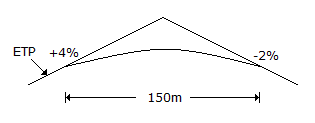# Civil Engineering - GATE Exam Questions - Discussion

### Discussion :: GATE Exam Questions - Section 4 (Q.No.41)

41.

A parabolic curve is used to connect a 4% upgrade with a 2% downgrade as shown in the below figure. The highest point on the summit is at a distance of (measured horizontally from the first tangent point - (FTP)[A]. 50 m [B]. 60 m [C]. 75 m [D]. 100 m

Explanation:

No answer description available for this question.

 Mashal Jins.M said: (Oct 24, 2015) Please any one explain that.

 Sumit said: (Feb 4, 2016) = (L*n)/n. = (150*4)/6. = 100 m.

 Tilahun.M said: (Feb 16, 2017) x = L * g1/A, = 150 * 4/6. = 100m.

 M .Suna said: (Jun 20, 2017) The correct Answer should be 100.

 Jagdish said: (Jul 6, 2017) I think 100 is the Answer.

 Ruchi Sinha said: (Jul 21, 2017) X= nL/N= 4 * 150/6 = 100.

 Vinay said: (Aug 30, 2017) x=n1.L/N = 100.

 Shivaraj said: (Jan 30, 2019) Yes, 100m is correct.

 Yesika said: (Jun 21, 2019) 100 m is correct one.